Published

# Arduino HID Media Controller

Can control media in pc by distance with come knobs and switches

IntermediateFull instructions provided1 hour907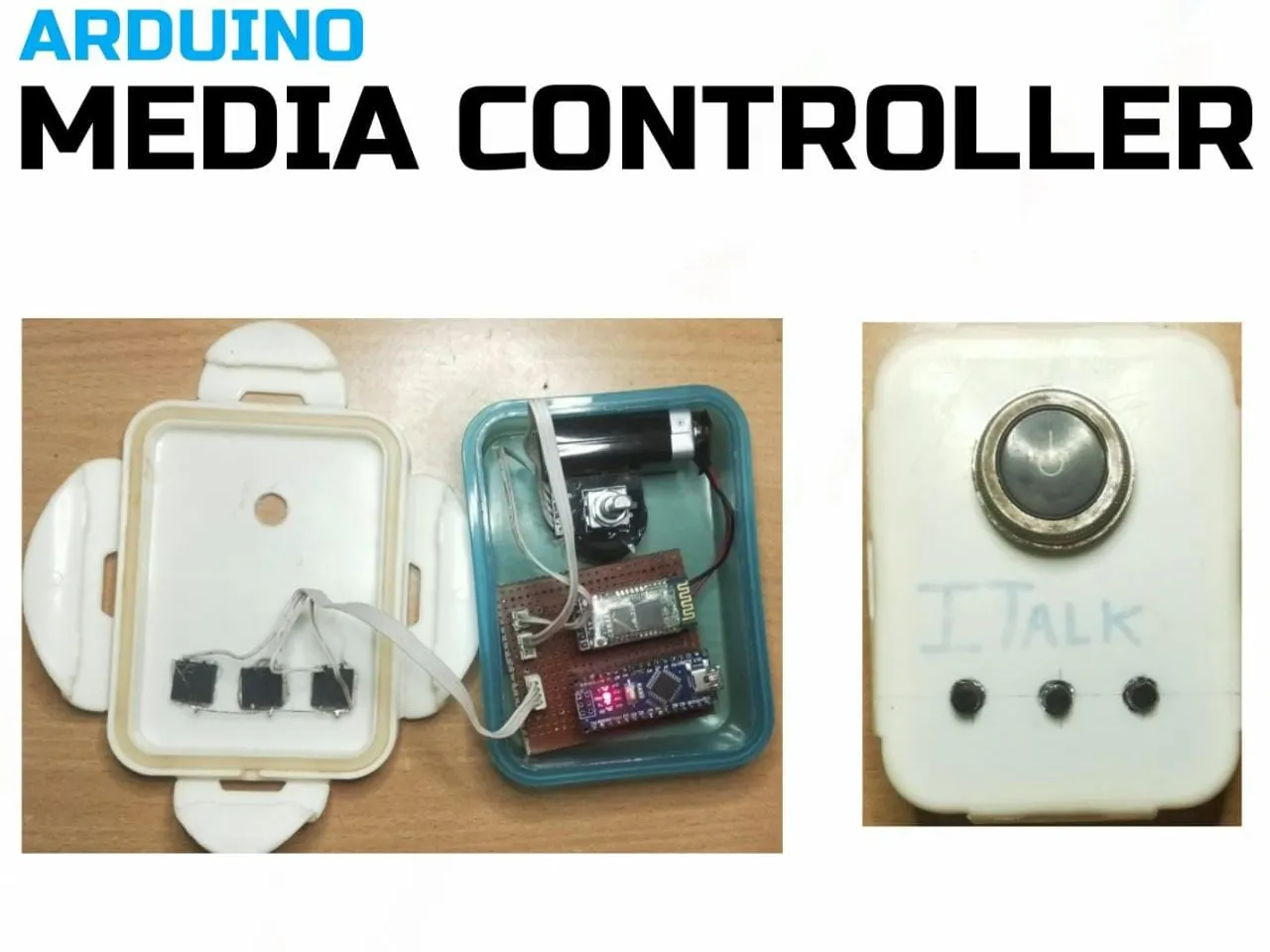## Things used in this project

### Hardware components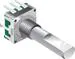Rotary Encoder with Push-Button
×1SparkFun Pushbutton switch 12mm
×3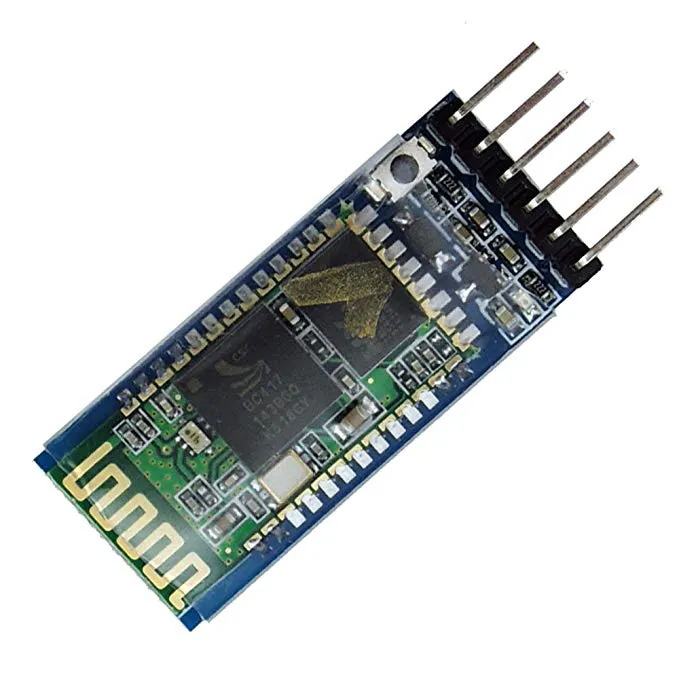HC-05 Bluetooth Module
×1Arduino Nano R3
×1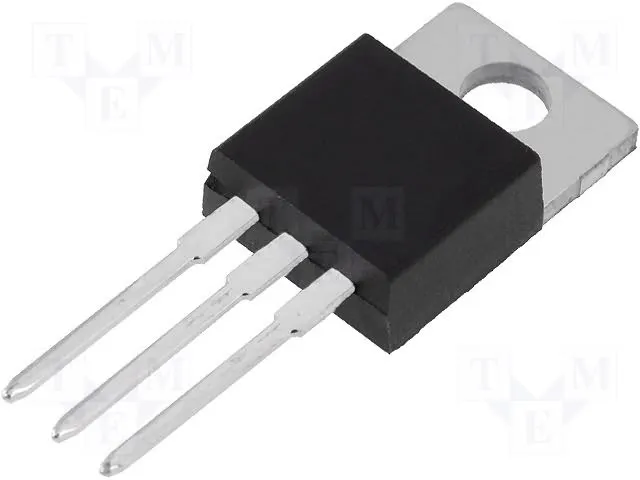Linear Regulator (7805)
×1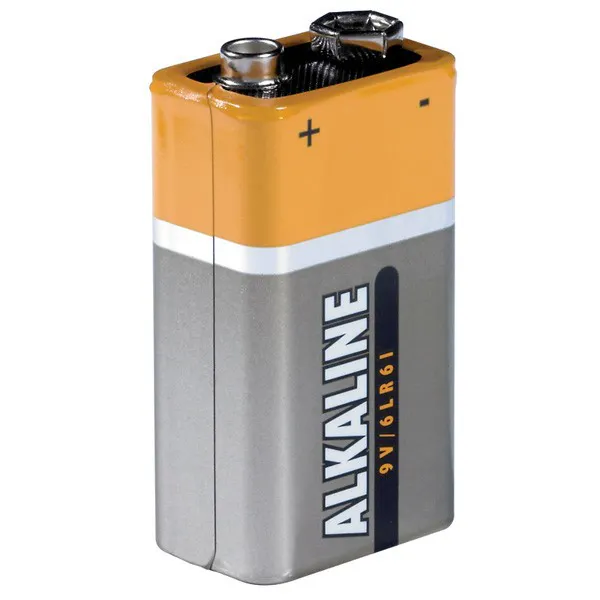9V battery (generic)
×1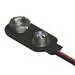9V Battery Clip
×1Jumper wires (generic)
×1

### Software apps and online servicesArduino IDE

### Hand tools and fabrication machinesSoldering iron (generic)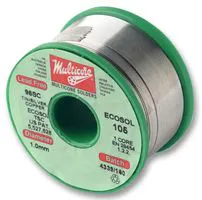Hot glue gun (generic)

## Schematics

### circuit diagram

if you're are following this so just remove pullup from Arduino code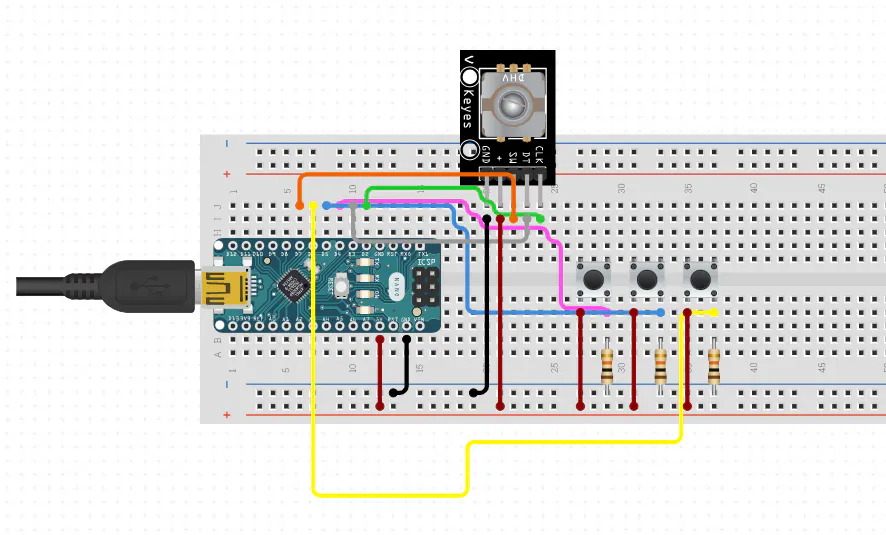## Code

### Arduino code

Arduino
```#define CLK 6
#define DT 4
float presslen = 0;
float presslen1 = 0;
int one1 = 100;
int two2 = 2000;
int btn = 8;
int btn2 = 9;
int btn3 = 7;
int btn4 = 5;
int counter = 0;
int currentCLK;
int previousCLK;

void setup() {
pinMode (CLK,INPUT);
pinMode (DT,INPUT);
pinMode (btn,INPUT_PULLUP);
pinMode (btn2,INPUT_PULLUP);
pinMode (btn3,INPUT_PULLUP);
pinMode (btn4,INPUT_PULLUP);// button on encdir
Serial.begin (9600);
}
void loop() {
if (currentCLK != previousCLK){
counter --;
delay(100);
Serial.println("N");
} else{
counter ++;
delay(100);
Serial.println("P");
}
}

delay(100);
presslen = presslen + 100;
}
delay(100);
presslen1 = presslen1 + 100;
}
if (presslen >= two2){
Serial.println("B");// for BASS FILTER
}
else if(presslen >= one1){
Serial.println("S");// for pause
}
if (presslen1 >= two2){
Serial.println("Z");
}
else if(presslen1 >= one1){
Serial.println("O");
}
Serial.println("F");
delay(500);// for forward
}
Serial.println("M");
delay(500);// for MUTE
}
presslen = 0;
presslen1 = 0;
//Serial.println("TEST PASS");
}
```

### Python code

Python
```import serial
import time
import webbrowser
import pyautogui
m5 = serial.Serial('COM5', 9600, timeout=.1)
while True:
if da == b'P':
pyautogui.press('volumeup')
elif da == b'N':
pyautogui.press('volumedown')
elif da == b'M':
pyautogui.press('volumemute')
elif da == b'O':
pyautogui.keyDown('shift')
pyautogui.press('p')
pyautogui.keyUp('shift')
elif da == b'Z':
elif da == b'S':
pyautogui.press('k')
elif da == b'F':
pyautogui.keyDown('shift')
pyautogui.press('n')
pyautogui.keyUp('shift')
elif da == b'B':
pyautogui.click(x=1261, y=1052)
time.sleep(2)
pyautogui.click(x=1427, y=95)
time.sleep(1)
pyautogui.click(x=1376, y=479)
time.sleep(1)
pyautogui.click(x=1352, y=762)
time.sleep(1)
pyautogui.click(x=62, y=535)
```

## Credits

### Amaan Javed

3 projects • 3 followers
Student